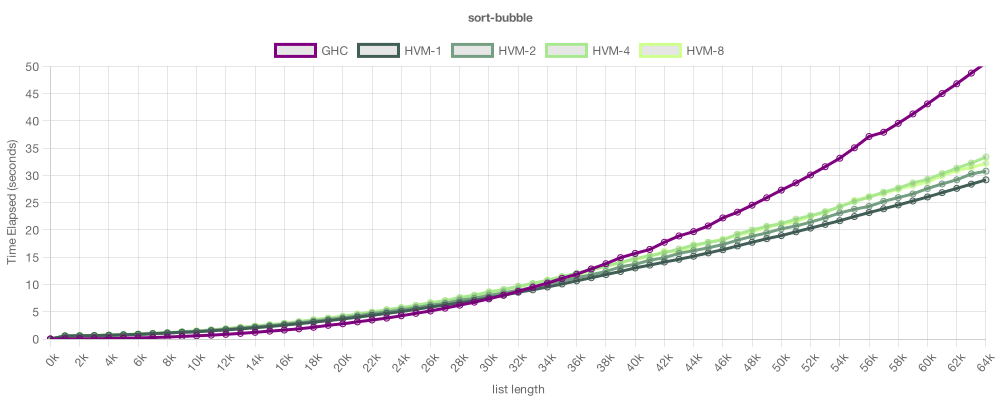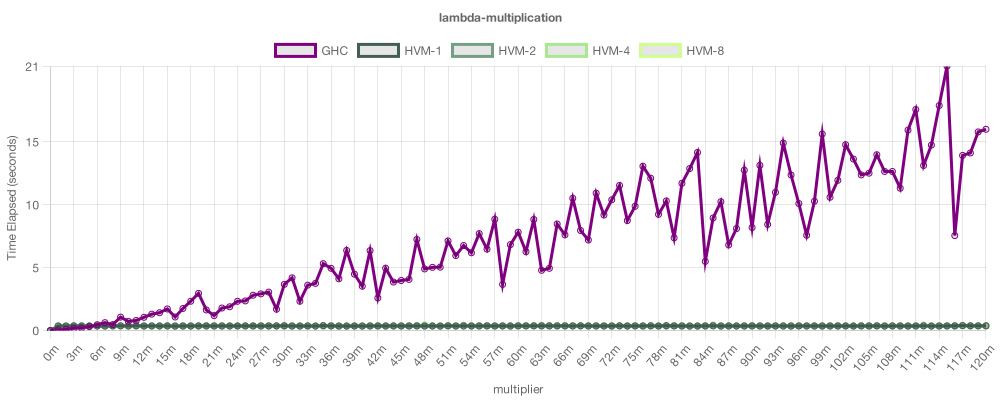## nightly bin+lib hvm

A massively parallel functional runtime

### 101 releases(1 stable)

 1.0.20-beta Dec 27, 2022 Nov 23, 2022 Jan 12, 2023 Oct 7, 2022 Mar 27, 2022

Used in 4 crates (3 directly)

560KB
6.5K SLoC

# High-order Virtual Machine (HVM)

High-order Virtual Machine (HVM) is a pure functional runtime that is lazy, non-garbage-collected and massively parallel. It is also beta-optimal, meaning that, for higher-order computations, it can be exponentially faster than alternatives, including Haskell's GHC.

That is possible due to a new model of computation, the Interaction Net, which supersedes the Turing Machine and the Lambda Calculus. Previous implementations of this model have been inefficient in practice, however, a recent breakthrough has drastically improved its efficiency, resulting in the HVM. Despite being relatively new, it already beats mature compilers in many cases, and is set to scale towards uncharted levels of performance.

Welcome to the massively parallel future of computers!

# Examples

Essentially, HVM is a minimalist functional language that is compiled to a novel runtime based on Interaction Nets. This approach is not only memory-efficient (no GC needed), but also has two significant advantages: automatic parallelism and beta-optimality. The idea is that you write a simple functional program, and HVM will turn it into a massively parallel, beta-optimal executable. The examples below highlight these advantages in action.

## Bubble Sort

 From: HVM/examples/sort/bubble/main.hvm From: HVM/examples/sort/bubble/main.hs ``````// sort : List -> List (Sort Nil) = Nil (Sort (Cons x xs)) = (Insert x (Sort xs)) // Insert : U60 -> List -> List (Insert v Nil) = (Cons v Nil) (Insert v (Cons x xs)) = (SwapGT (> v x) v x xs) // SwapGT : U60 -> U60 -> U60 -> List -> List (SwapGT 0 v x xs) = (Cons v (Cons x xs)) (SwapGT 1 v x xs) = (Cons x (Insert v xs)) `````` ``````sort' :: List -> List sort' Nil = Nil sort' (Cons x xs) = insert x (sort' xs) insert :: Word64 -> List -> List insert v Nil = Cons v Nil insert v (Cons x xs) = swapGT (if v > x then 1 else 0) v x xs swapGT :: Word64 -> Word64 -> Word64 -> List -> List swapGT 0 v x xs = Cons v (Cons x xs) swapGT 1 v x xs = Cons x (insert v xs) ``````On this example, we run a simple, recursive Bubble Sort on both HVM and GHC (Haskell's compiler). Notice the algorithms are identical. The chart shows how much time each runtime took to sort a list of given size (the lower, the better). The purple line shows GHC (single-thread), the green lines show HVM (1, 2, 4 and 8 threads). As you can see, both perform similarly, with HVM having a small edge. Sadly, here, its performance doesn't improve with added cores. That's because Bubble Sort is an inherently sequential algorithm, so HVM can't improve it.

 From: HVM/examples/sort/radix/main.hvm From: HVM/examples/sort/radix/main.hs ``````// Sort : Arr -> Arr (Sort t) = (ToArr 0 (ToMap t)) // ToMap : Arr -> Map (ToMap Null) = Free (ToMap (Leaf a)) = (Radix a) (ToMap (Node a b)) = (Merge (ToMap a) (ToMap b)) // ToArr : Map -> Arr (ToArr x Free) = Null (ToArr x Used) = (Leaf x) (ToArr x (Both a b)) = let a = (ToArr (+ (* x 2) 0) a) let b = (ToArr (+ (* x 2) 1) b) (Node a b) // Merge : Map -> Map -> Map (Merge Free Free) = Free (Merge Free Used) = Used (Merge Used Free) = Used (Merge Used Used) = Used (Merge Free (Both c d)) = (Both c d) (Merge (Both a b) Free) = (Both a b) (Merge (Both a b) (Both c d)) = (Both (Merge a c) (Merge b d)) `````` ``````sort :: Arr -> Arr sort t = toArr 0 (toMap t) toMap :: Arr -> Map toMap Null = Free toMap (Leaf a) = radix a toMap (Node a b) = merge (toMap a) (toMap b) toArr :: Word64 -> Map -> Arr toArr x Free = Null toArr x Used = Leaf x toArr x (Both a b) = let a' = toArr (x * 2 + 0) a b' = toArr (x * 2 + 1) b in Node a' b' merge :: Map -> Map -> Map merge Free Free = Free merge Free Used = Used merge Used Free = Used merge Used Used = Used merge Free (Both c d) = (Both c d) merge (Both a b) Free = (Both a b) merge (Both a b) (Both c d) = (Both (merge a c) (merge b d)) ``````On this example, we try a Radix Sort, based on merging immutable trees. In this test, for now, single-thread performance was superior on GHC - and this is often the case, since GHC is much older and has astronomically more micro-optimizations - yet, since this algorithm is inherently parallel, HVM was able to outperform GHC given enough cores. With 8 threads, HVM sorted a large list 2.5x faster than GHC.

Keep in mind one could parallelize the Haskell version with `par` annotations, but that would demand time-consuming, expensive refactoring - and, in some cases, it isn't even possible to use all the available parallelism with `par` alone. HVM, on the other hands, will automatically distribute parallel workloads through all available cores, achieving horizontal scalability. As HVM matures, the single-thread gap will decrease significantly.

## Lambda Multiplication

 From: HVM/examples/lambda/multiplication/main.hvm From: HVM/examples/lambda/multiplication/main.hs ``````// Increments a Bits by 1 // Inc : Bits -> Bits (Inc xs) = λex λox λix let e = ex let o = ix let i = λp (ox (Inc p)) (xs e o i) // Adds two Bits // Add : Bits -> Bits -> Bits (Add xs ys) = (App xs λx(Inc x) ys) // Multiplies two Bits // Mul : Bits -> Bits -> Bits (Mul xs ys) = let e = End let o = λp (B0 (Mul p ys)) let i = λp (Add ys (B0 (Mul p ys))) (xs e o i) `````` ``````-- Increments a Bits by 1 inc :: Bits -> Bits inc xs = Bits \$ \ex -> \ox -> \ix -> let e = ex o = ix i = \p -> ox (inc p) in get xs e o i -- Adds two Bits add :: Bits -> Bits -> Bits add xs ys = app xs (\x -> inc x) ys -- Muls two Bits mul :: Bits -> Bits -> Bits mul xs ys = let e = end o = \p -> b0 (mul p ys) i = \p -> add ys (b0 (mul p ys)) in get xs e o i ``````This example implements bitwise multiplication using λ-encodings. Its purpose is to show yet another important advantage of HVM: beta-optimality. This chart isn't wrong: HVM multiplies λ-encoded numbers exponentially faster than GHC, since it can deal with very higher-order programs with optimal asymptotics, while GHC can not. As esoteric as this technique may look, it can actually be very useful to design efficient functional algorithms. One application, for example, is to implement runtime deforestation for immutable datatypes. In general, HVM is capable of applying any fusible function `2^n` times in linear time, which sounds impossible, but is indeed true.

# Getting Started

1. Install Rust nightly:

``````curl --proto '=https' --tlsv1.2 -sSf https://sh.rustup.rs | sh
rustup default nightly
``````
2. Install HVM:

``````cargo install hvm
``````
3. Run an HVM expression:

``````hvm run "(@x(+ x 1) 41)"
``````

That's it! For more advanced usage, check the complete guide.